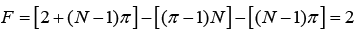Courses

# Derivation of the Phase Rule Civil Engineering (CE) Notes | EduRev

## Civil Engineering (CE) : Derivation of the Phase Rule Civil Engineering (CE) Notes | EduRev

The document Derivation of the Phase Rule Civil Engineering (CE) Notes | EduRev is a part of the Civil Engineering (CE) Course Thermodynamics.
All you need of Civil Engineering (CE) at this link: Civil Engineering (CE)

Derivation of the Phase Rule

The phase rule was introduced in section 1.5 without proof. Here we develop its mathematical form based on the tenets of solution thermodynamics and phase equilibrium criterion presented in the last chapter. Consider a non-reactive system under equilibrium, with π phases each containing N independent chemical species. The degrees of freedom for the system, i.e., the number of intensive variables that may vary independently of each other would be given by:

Degrees of freedom = Total number of systemic intensive variables –number of independent equations relating all the variables.

For the system of interest here the above terms are as follows: I. Total number of systemic intensive variables (also called the phase rule variables)= T, P and (N-1) species mole fractions for each of the π phases II. Number of independent relations connecting the phase rule variables = (π − 1) N

The second relation above follows from the fact that for each of the N species one may use the chemical potential equality relation across all π phases, as described by eqn. 6.52. It follows that for each component there can be only (π − 1) independent relations. Thus the phase rule may be rewritten as:

F = [ 2 + ( N − 1)π ] − [(π − 1) N ] = 2 + N−π                            ......(7.18)

It may be noted that the actual mass of each of the species present are not considered as phase rule variables, as they cannot influence the intensive state of the system. A special case of the phase rule obtains for closed systems for which the initial mass for each species is fixed. Since no mass can enter or leave the system, the extensive state of the system is rendered fixed along with the intensive variables. Therefore, apart from the (π − 1) N constraining relations involving the species chemical potentials, there is an additional [( N − 1)π ] constraint on the mass of each species; this follows from the fact that if a quantum of a species leaves a phase it must reappear in another or more. Thus the phase rule eqn. leads to:......(7.19)

The above equation is known as the Duhem's theorem. It implies that for any closed system formed initially from given masses of a number of chemical species, the equilibrium state is completely determined when any two independent variables are fixed. The two independent variables that one may choose to specify may be either intensive or extensive. However, the number of independent intensive variables is given by the phase rule. Therefore, it follows that when F = 1, at least one of the two variables must be extensive, and when F = 0, both must be extensive.

Offer running on EduRev: Apply code STAYHOME200 to get INR 200 off on our premium plan EduRev Infinity!

## Thermodynamics

56 videos|92 docs|33 tests

,

,

,

,

,

,

,

,

,

,

,

,

,

,

,

,

,

,

,

,

,

;